# A block with mass m = 5.8 kg is attached to two springs with spring constants kleft = 37 N/m and Kright = 55 N/m. T...A block with mass m = 5.8 kg is attached to two springs with spring constants kleft = 37 N/m and Kright = 55 N/m. The block is pulled a distance x = 0.24 m to the left of its equilibrium position and released from rest.

1) What is the magnitude of the net force on the block (the moment it is released)?

2) What is the effective spring constant of the two springs?

3) What is the period of oscillation of the block?

4) How long does it take the block to return to equilibrium for the first time?

5) What is the speed of the block as it passes through the equilibrium position?

6) What is the magnitude of the acceleration of the block as it passes through equilibrium?

7) Where is the block located, relative to equilibrium, at a time 0.92 s after it is released? (if the block is left of equilibrium give the answer as a negative value; if the block is right of equilibrium give the answer as a positive value)

8) What is the net force on the block at this time 0.92 s? (a negative force is to the left; a positive force is to the right)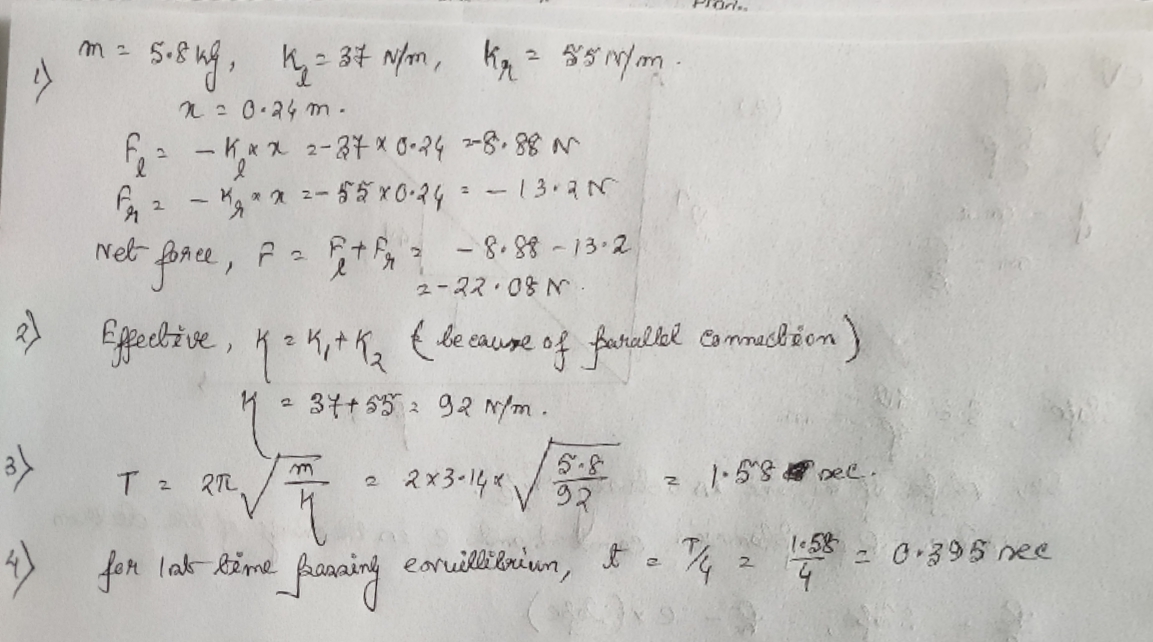#### Earn Coin

Coins can be redeemed for fabulous gifts.

Similar Homework Help Questions
• ### A block with mass m = 5.7 kg is attached to two springs with spring constants...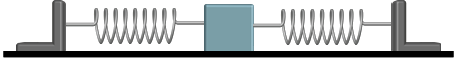A block with mass m = 5.7 kg is attached to two springs with spring constants kleft = 36 N/m and kright = 53 N/m. The block is pulled a distance x = 0.23 m to the left of its equilibrium position and released from rest. 1) What is the magnitude of the net force on the block (the moment it is released)?  2) What is the effective spring constant of the two springs?  3) What is the period of oscillation of...

• ### A block with mass m = 6.2 kg is attached to two springs with spring constants...A block with mass m = 6.2 kg is attached to two springs with spring constants kleft = 31 N/m and kright = 49 N/m. The block is pulled a distance x = 0.2 m to the left of its equilibrium position and released from rest. 1) What is the magnitude of the net force on the block (the moment it is released)? N 2) What is the effective spring constant of the two springs? N/m 3) What is the...

• ### A block with mass m = 7 kg is attached to two springs with spring constants...

A block with mass m = 7 kg is attached to two springs with spring constants kleft = 37 N/m and kright = 48 N/m. The block is pulled a distance x = 0.21 m to the left of its equilibrium position and released from rest. 6)What is the magnitude of the acceleration of the block as it passes through equilibrium? 7)Where is the block located, relative to equilibrium, at a time 1.23 s after it is released? (if the...

• ### A block with mass m = 7.4 kg is attached to two springs with spring constants...A block with mass m = 7.4 kg is attached to two springs with spring constants kleft = 31 N/m and kright = 53 N/m. The block is pulled a distance x = 0.27 m to the left of its equilibrium position and released from rest. 7) Where is the block located, relative to equilibrium, at a time 1.04 s after it is released? (if the block is left of equilibrium give the answer as a negative value; if the...

• ### Standard Exe Mass On A block with mass m - 7.2 kg is attached to two springs with spring constants kleft -29 N/m and Kright-51 N/m. The block is pulled a distance x 0.24 m to the left of its equilibr...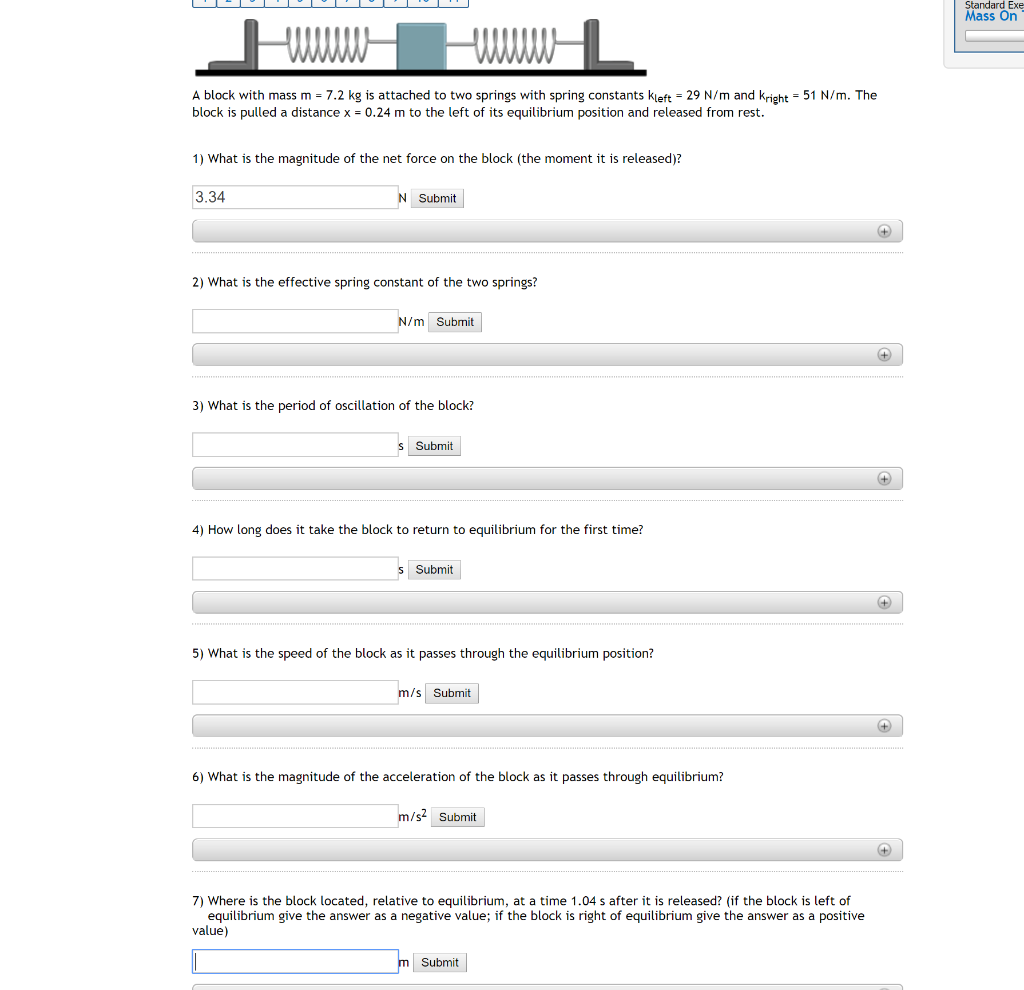Standard Exe Mass On A block with mass m - 7.2 kg is attached to two springs with spring constants kleft -29 N/m and Kright-51 N/m. The block is pulled a distance x 0.24 m to the left of its equilibrium position and released from rest. 1) What is the magnitude of the net force on the block (the moment it is released)? 3.34 Submit 2) What is the effective spring constant of the two springs? N/m Submit 3) What...

• ### A block with mass m = 7.2 kg is attached to two springs with spring constants...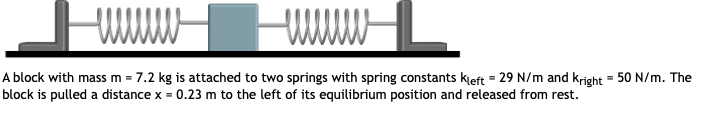A block with mass m = 7.2 kg is attached to two springs with spring constants kleft = 29 N/m and Kright = 50 N/m. The block is pulled a distance x = 0.23 m to the left of its equilibrium position and released from rest. 7) Where is the block located, relative to equilibrium, at a time 1.31 s after it is released? (if the block is left of equilibrium give the answer as a negative value; if the...

• ### Simple Harmonic Motion - block & spring

A block with mass m = 4.6 kg is attached to two springs with spring constants kleft = 31 N/m and kright = 55 N/m. The block is pulled a distance x = 0.2 m to the leftof its equilibrium position and released from rest.Find the time it takes to reach equilibrium position the first time, and the speed and magnitude of acceleration as it passes through equilibrium.Where is the block located, relative to equilibrium, at a time 1.02 s...

• ### 3, A block of mass m = 2.00 kg is attached to a spring of force...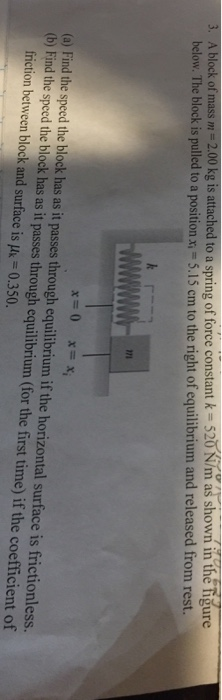3, A block of mass m = 2.00 kg is attached to a spring of force constant k = 520 N/m as shown in the figure low. The block is pulled to a position ax, -5.15 em to the right of equilibrium and released from rest. x=0 x=x o Find the sped he lodk has as it passes through equililbrium if the horizontal surface is frctionless (b) Find the speed the block has as it passes through equilibrium (for the...

• ### A mass of 500 grams is attached to two springs whose spring constants are k1=2 N/m...

A mass of 500 grams is attached to two springs whose spring constants are k1=2 N/m and k2 = 5 N/m, which are in turn attached to a wall. The system is on a horizontal frictionless surface. The system is displaced to the right and released. (a) What is the effective spring constant of the two springs in ”series”? Hint use Hooke’s law and the fact that the force required to displace the system is the same acting on each...

• ### A block of mass m = 6.04 kg is attached to a spring with spring constant...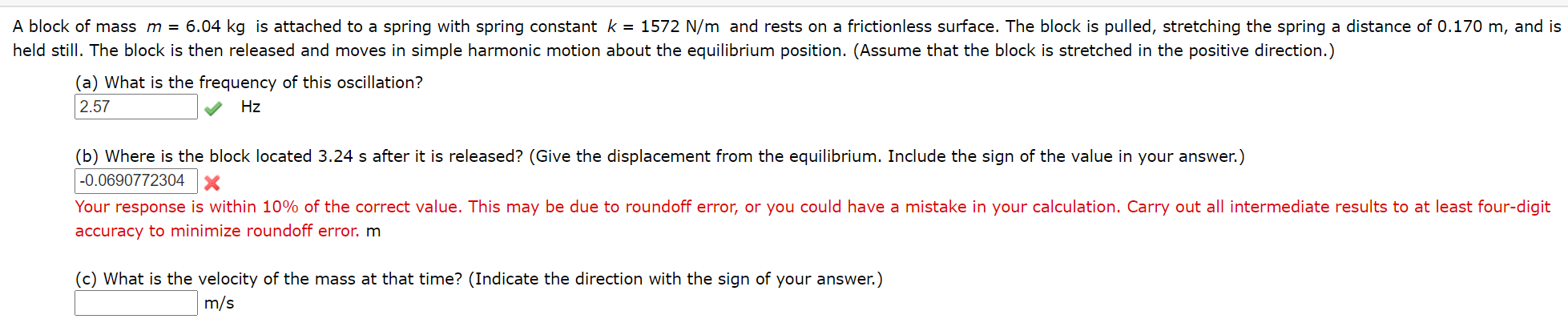A block of mass m = 6.04 kg is attached to a spring with spring constant k = 1572 N/m and rests on a frictionless surface. The block is pulled, stretching the spring a distance of 0.170 m, and is held still. The block is then released and moves in simple harmonic motion about the equilibrium position. (Assume that the block is stretched in the positive direction.) (a) What is the frequency of this oscillation? 2.57 Hz (b) Where is...